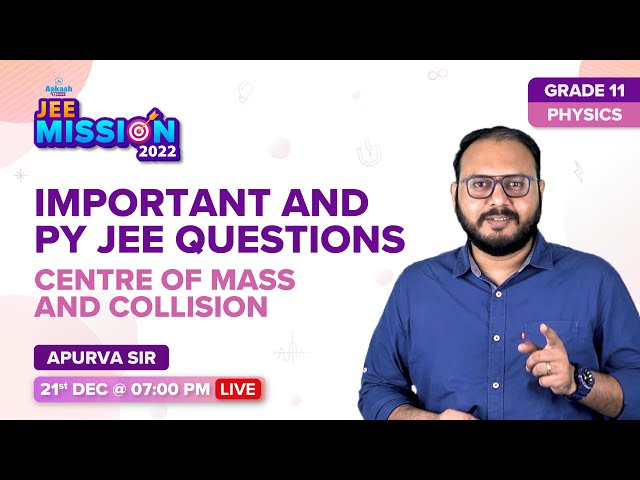Win up to 100% scholarship on Aakash BYJU'S JEE/NEET courses with ABNAT Win up to 100% scholarship on Aakash BYJU'S JEE/NEET courses with ABNAT

# JEE Advanced Previous Year Questions with Solutions on Centre of Mass

The centre of mass of a body or system of a particle is defined as a point at which the whole of the mass of the body or all the masses of a system of particle appeared to be concentrated. In physics, we can say that the centre of mass is a point at the centre of the distribution of mass in space (also known as balance point) wherein the weighted relative position of the distributed mass has a sum of zero. In simple words, the centre of mass is a position that is relative to an object. We can say that it is the average position of all the parts of the system or it is the mean location of a distribution of mass in space. It is a point where force is usually applied that results in linear acceleration without any angular acceleration.

Question 1) A particle of mass moving with a speed v collide elastically with the end of a uniform rod of mass M and length L perpendicularly as shown in the figure. If the particle comes to rest after collision, find the value of m/M.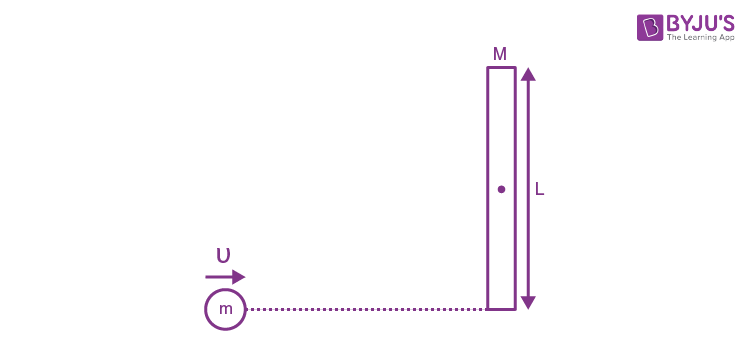(A) 1/3

(B) 1/2

(C) 1/4

(D) 1/5

Solution: (A) 1/3

Applying conservation of angular momentum about the Centre of mass of rod

$$\begin{array}{l}mv\left ( \frac{L}{2} \right )=M\left ( \frac{L^{2}}{12} \right )\omega\end{array}$$

ω = 6mu/ML

Conservation of Kinetic Energy

(1/2)mu2 = (1/2)(ML2/12)ω2

mu2 = (ML2/12)ω2

mu2 = (ML2/12)[36m2u2/M2L2]

⇒ m/M = 1/3

Question 2) A horizontal force F is applied at the center of mass of a cylindrical object of mass m and radius R, perpendicular to its axis as shown in the figure. The coefficient of friction between the object and the ground is μ . The center of mass of the object has an acceleration a. The acceleration due to gravity is g. Given that the object rolls without slipping, which of the following statement(s) is(are) correct?

(A) For the same F, the value of a does not depend on whether the cylinder is solid or hollow

(B) For a solid cylinder, the maximum possible value of a is 2μg

(C) The magnitude of the frictional force on the object due to the ground is always μmg

(D) For a thin-walled hollow cylinder, a = F/2m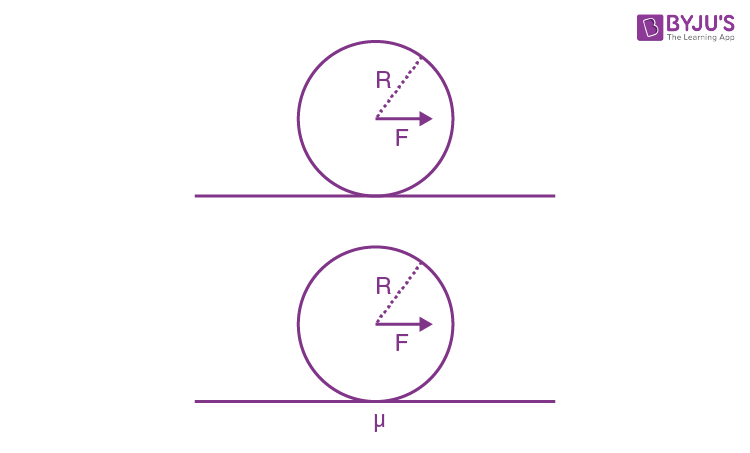Solution:

For solid cylinder,

$$\begin{array}{l}F\times R= \frac{3}{2}mR^{2}\times \frac{a}{R}\end{array}$$

⇒ a = 2F/3m

For hollow cylinder,

$$\begin{array}{l}F\times R= 2mR^{2}\times \frac{a}{R}\end{array}$$

⇒ a = F/3m

For solid cylinder

f = F – m x (2F/3m) = (F/3) ≤ μmg

⇒ F≤ 3μmg

Therefore,

a ≤ (2/3m) x (3μmg)

⇒ amax = 2μg

Question 3) The distance between two stars of masses 3MS and 6MS is 9R. Here R is the mean distance between the centres of the Earth and the Sun, and MS is the mass of the Sun. The two stars orbit around their common centre of mass in circular orbits with period nT, where T is the period of Earth’s revolution around the Sun. The value of n is ___.

Solution: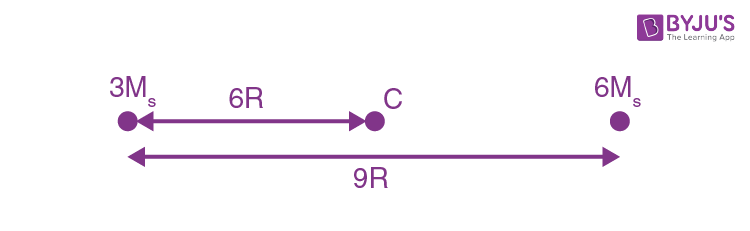Centre of mass of system lies at 6R from lighter mass

$$\begin{array}{l}\left [ 3M_{s}\omega ^{2}\times 6R \right ]=\frac{G(18M^{2}_{s})}{81R^{2}}\end{array}$$
$$\begin{array}{l}\omega ^{2} = \frac{GM}{81R^{3}}\end{array}$$

T’ = 2π/ω

$$\begin{array}{l}T^{‘}=2π\sqrt{\frac{81R^{3}}{GM_{s}}}\end{array}$$

T’ = 9T

n = 9

Question 4) Three-point particles of masses 1.0 kg, 1.5 kg and 2.5 kg are placed at three corners of a right angle triangle of sides 4.0 cm, 3.0 cm and 5.0 cm as shown in the figure. The centre of mass of the system is at a point: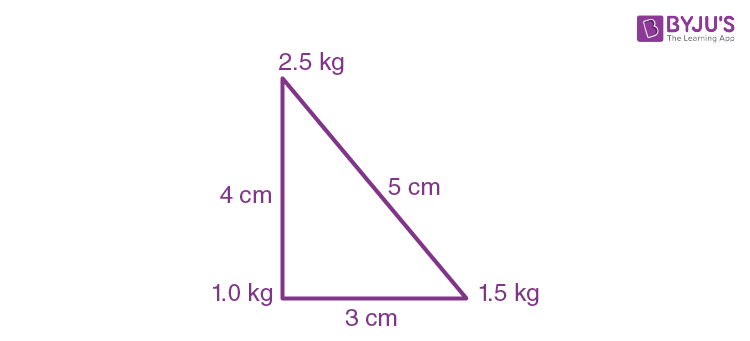(A) 0.6 cm right and 2.0 cm above 1 kg mass

(B) 1.5 cm right and 1.2 cm above 1 kg mass

(C) 2.0 cm right and 0.9 cm above 1 kg mass

(D) 0.9 cm right and 2.0 cm above 1 kg mass

Answer: (D) 0.9 cm right and 2.0 cm above 1 kg mass

Solution: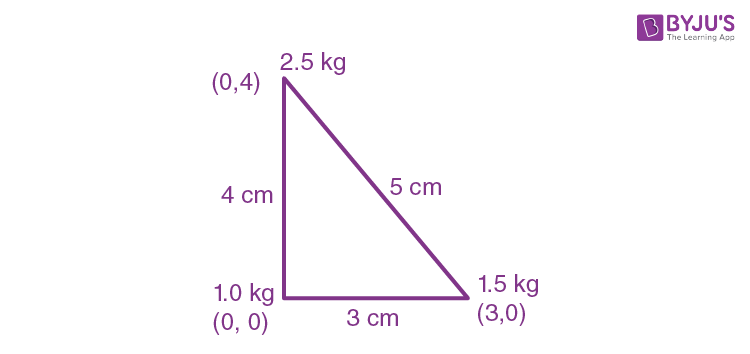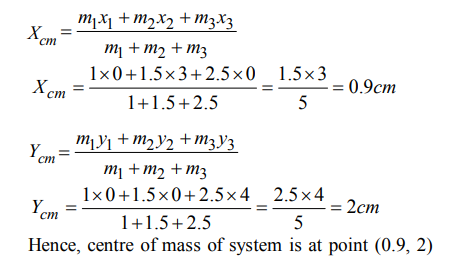Question 5) A uniform rectangular thin sheet ABCD of mass M has length a and breath b, as shown in the figure. If the shaded portion HBGO is cut-off, the coordinates of the centre of mass of the remaining portion will be: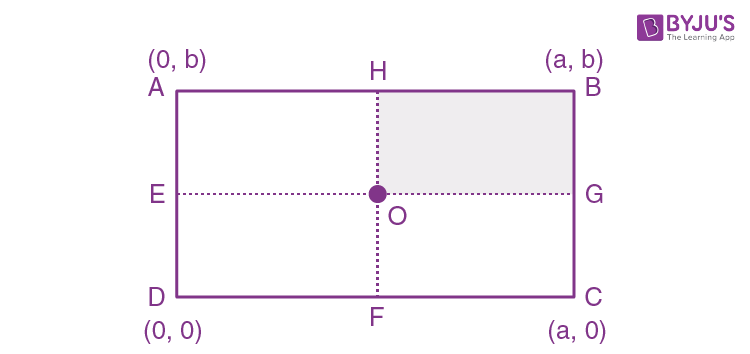$$\begin{array}{l}(A)\ \left [ \frac{3a}{4},\frac{3b}{4} \right ]\end{array}$$
$$\begin{array}{l}(B)\ \left [ \frac{5a}{3},\frac{5b}{3} \right ]\end{array}$$
$$\begin{array}{l}(C)\ \left [ \frac{2a}{3},\frac{2b}{3} \right ]\end{array}$$
$$\begin{array}{l}(D)\ \left [ \frac{5a}{12},\frac{5b}{12} \right ]\end{array}$$

$$\begin{array}{l}\text{Answer}: (D)\ \left [ \frac{5a}{12},\frac{5b}{12} \right ]\end{array}$$

Solution:

From the figure

x1 = a/4

x2 = a/4

x3 = 3a/4

xcom = (m1x1 + m2x2 + m3x3)/(m1 + m2 + m3)

= (m(a/4) + m(a/4) + m(3a/4))/(m + m + m)

= 5a/12

y1 = 3b/4

y2 = b/4

y3 = b/4

ycom = (m1y1 + m2y2 + m3y3)/(m1 + m2 + m3)

= (m(3b/4) + m(b/4) + m(b/4))/(m + m + m)

= 5b/12

(xcom, ycom) = [5a/12, 5b/12]

Question 6) The coordinates of the centre of mass of a uniform flag-shaped lamina of mass 4 Kg. (The coordinates of the same are shown in figure) are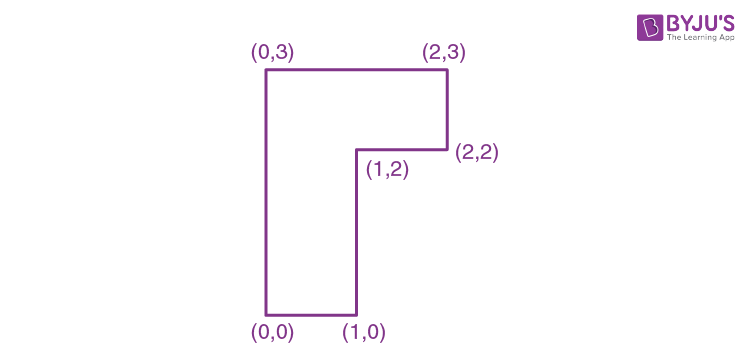(A) (1.25 m, 1.50 m)

(B) (0.75 m, 1.75 m)

(C) (0.75 m, 0.75 m)

(D) (1 m, 1.75 m)

Answer: (B) (0.75 m, 1.75 m)

Solution: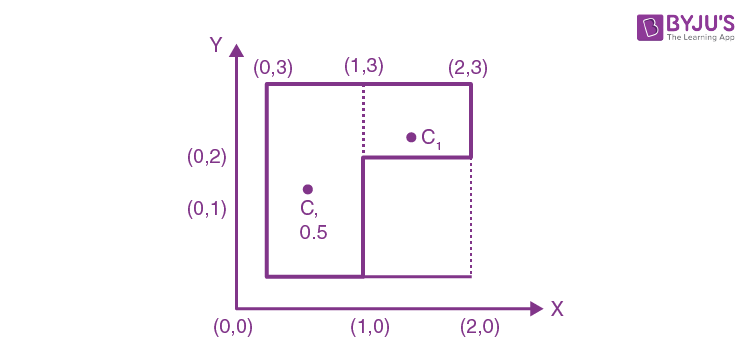For given lamina

m1 = 1, C1 = (1.5, 2.5)

m2 = 3, C2 = (0.5, 1.5)

$$\begin{array}{l}X_{cm}=\frac{m_{1}x_{1}+m_{2}x_{2}}{m_{1}+m_{2}}=\frac{1.5+1.5}{4}= 0.75\end{array}$$
$$\begin{array}{l}Y_{cm}=\frac{m_{1}y_{1}+m_{2}y_{2}}{m_{1}+m_{2}}=\frac{2.5+4.5}{4}= 1.75\end{array}$$

Therefore, the coordinates of the centre of mass of flag-shaped lamina is (0.75, 1.75)

Question 7) Two particles A and B initially at rest, move towards each other under the mutual force of attraction. At the instant when the speed of A is V and the speed of B is 2V, the speed of the centre of mass of the system is

(A) 3 V

(B) V

(C)1.5 V

(D) zero

Solution:

Net external force on system is zero. Therefore centre of mass always remains at rest.

Question 8) A rod of weight w is supported by two parallel knife edges A and B and is in equilibrium in a horizontal position. The knives are at a distance d each other. The centre of mass of the rod is at distance x from A. The normal reaction on A is ______

(A) W(d – x)/d

(B) Wx/d

(C) Wd/s

(D) W(d – x)/x

Solution: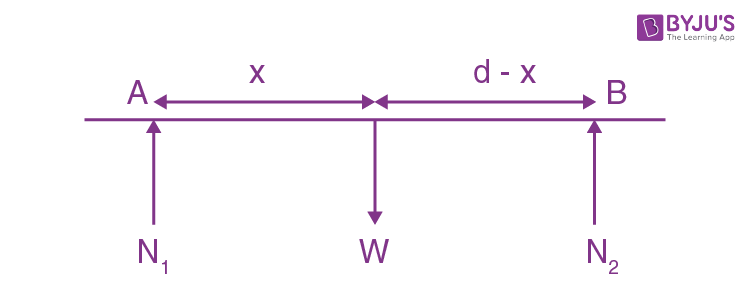N1 = normal reaction on A

N2 = Normal reaction on B

W = weight of the rod

In vertical equilibrium,

N1 + N2 = W ——–(1)

Torque balance about centre of mass of the rod

N1x = N2(d – x)

Putting the value of N2 from equation (1)

N1x = (W – N1)(d – x)

⇒ N1x = Wd – Wx – N1d + N1x

N1 = W(d – x)/d

Question 9) Four particles A,B,C and D with masses mA = m, mB = 2m, mc = 3m and mD = 4m are at the corners of the square. They have accelerations of equal magnitude with directions as shown. The acceleration of the centre of mass of the particles is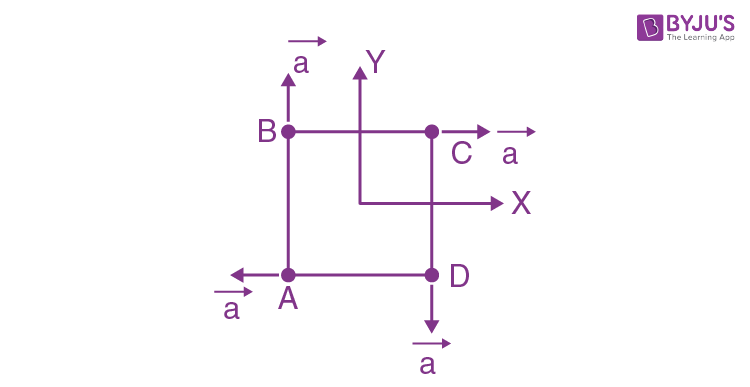$$\begin{array}{l}(A)\ \frac{a}{5}(\hat{i}-\hat{j})\end{array}$$

(B) a

(C) Zero

$$\begin{array}{l}(D)\ \frac{a}{5}(\hat{i}+\hat{j})\end{array}$$

Solution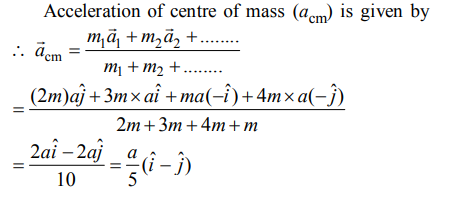Question 10) Distance of the centre of mass of a solid uniform cone from its vertex is z0. If the radius of its base is R and its height is h then z0 is equal to:

(A) 5h/8

(B) 3h2/8R

(C) h2/4R

(D) 3h/4

Solution: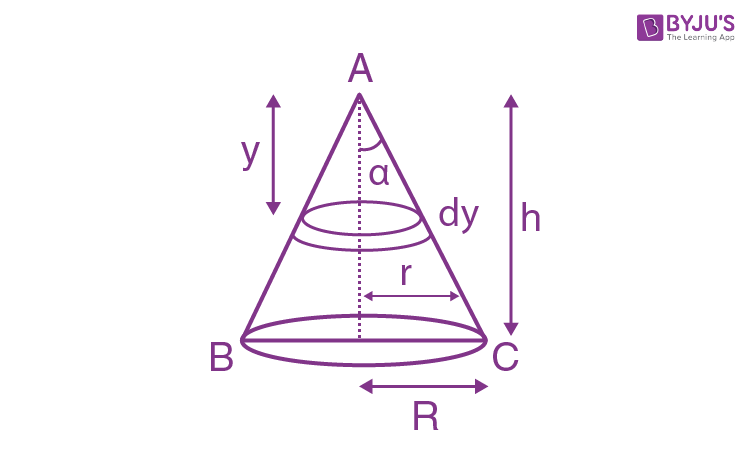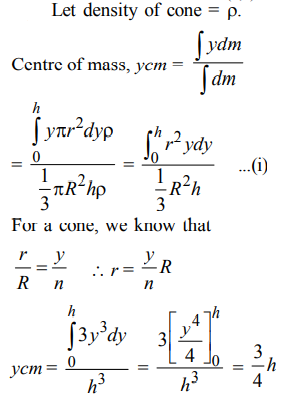## Centre of Mass and Collision JEE Main/Advanced Previous Year Questions